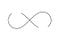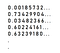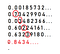# Some Infinities Are Bigger Than OthersImage from Creative Fabrica

You know, out of all the mind-bending things we like to say to try and get people interested in math (Look! This Mobius strip is an object with only one side), saying that “some infinities are bigger than others” has got to be one of the most popular and also one of the most mind-fucking to people who don’t know what you’re talking about. If you have no idea what I’m talking about either, with “types of infinity” and some being “bigger” than others and all that, I get it. Even though this is one of those astonishing, hall-of-fame moments in the math world, it sounds crazy.

Yes, there are different types of infinity. Some types of infinity are bigger than others. And I promise this will make sense by the end.

First off, what even is infinity exactly? We know that it means “really fucking big” but how is it actually defined? The way we use the term “infinity” is actually pretty vague, and this often confuses people. Is it a number, or a concept, or something else? Usually, we just use “infinity” as a label for some arbitrarily large value, because it turns out this concept of an arbitrarily large value shows up over and over in mathematics. But again, this label is kind of vague. We can do better and make the notion of “ridiculously big” more specific. This is where types of infinity come into play.

The set {1,2,3} of three positive whole numbers is a finite set because, well, the set is finite. There are only three numbers in there (the number of objects in a set is called the cardinality). Now the set of all positive whole numbers on the other hand, aka the natural numbers, is clearly infinite, because there’s no end to them. The set {1,2,3,4,5…} goes on forever. However… you might notice there’s a clear pattern to the numbers here. If I’m listing out the natural numbers, to get the next number in the sequence I can always just take the last number and add 1. The number 6 follows 5 in this set because 6=5+1. In other words, there’s a rule here.

Even though this is an infinite set, there’s a way for me to order all the numbers in this set according to some rule. In theory, if I had an infinite amount of time, I could use this rule to actually count all of these natural numbers. So this is called a countably infinite set.

Now I know what you’re thinking; But Mike, does this mean some sets are uncountably infinite? Yes. Yes exactly. This is called an uncountable set.

An uncountable set is so fucking colossal that you can’t even count the numbers in the set. An example of this would be the real numbers. The real numbers, to refresh your memory, include whole numbers, negative numbers, rational numbers (think fractions), and irrational numbers (think pi or the square root of 2).

We know that the positive whole numbers (the natural numbers) are countably infinite. The positive and negative whole numbers (aka the integers) are also countably infinite, because there’s still a way to count them. The order would look something like this: 0, 1, -1, 2, -2, 3, -3… There is still a way to count the integers.

However, there’s no possible method or rule to count the real numbers in this way. Even looking at the real numbers between 0 and 1, how would you start counting them? Would you start with… 0.0000001? Well what about 0.0000000001 instead? Hopefully it makes some intuitive sense here that the real numbers are a different kind of set in this way, that there’s no way to actually count the real numbers.

Georg Cantor went even farther and wrote formal proofs that the real numbers are uncountable, the most famous being Cantor’s diagonal argument. Here’s the rough idea: let’s say we want to show that the set of all real numbers between 0 and 1 is uncountable (this is true by the way). Let’s pretend we have listed all the numbers in this set, every single number between 0 and 1. This would look like a big list of decimals, many of which go on for ridiculous lengths. It would look something like this:big list of real numbers between 0 and 1

Cantor would then say “Oh yeah? That’s your list of all the real numbers between 0 and 1? I bet I can find a number that’s not in your list,” which would prove that there’s no way we can ever list (or count) all of these numbers. Cantor then slammed his drink on the table (I think) and said something like, “Make a new number which is equal to the first digit of the first number plus 1, and then the second digit of the second number plus 1, and so on until we’ve gotten through all the numbers in your list.” In other words we go down the diagonal of our list (That’s where the name comes from!) and modify each number by 1:our new number in red

Let’s call our new number “Dave.” Dave can not be in the list of numbers we made eariler. How do we know this? Well Dave is clearly different from the first number in our list, because we defined Dave as differing by the first number in that first digit. Similarly, Dave differs from every number in the list by at least one digit, because that’s how Dave is defined. So Dave can’t possibly be in the list, which would mean our “complete list” of all the real numbers between 0 and 1 was actually incomplete, making that set uncountable.

The set of all real numbers is uncountable too, and basically this means that the infinity we’ve used to describe the real numbers is a different kind of infinity than the kind used to describe the integers. The integers are infinite in a sense of practicality. We will never be able to list all the integers, and there is no “largest integer:” we can always find an integer larger than the last. On the other hand, the real numbers are infinite in a different way. The real numbers are infinite in the sense that trying to count them doesn’t even seem to apply to these numbers. Saying the real numbers are infinite is much grander in scope than saying the integers are infinite. And yes, while both the integers and the real numbers are infinite, the real numbers are much larger than the integers. One type of infinity is larger than another type of infinity.

So I guess the next time someone says “I love you a million,” and you say “I love you a billion,” and it keeps getting higher until they say “I love you infinity,” you can now respond with “I love you an uncountably infinite amount,” and watch the confusion on their face as you bask in the knowledge that you’re technically correct, thanks to your new understanding of math. Have a good one!

Math Tutor | Orange County, CA | If you want to reach out beneschan@gmail.com

## More from Mike Beneschan

Math Tutor | Orange County, CA | If you want to reach out beneschan@gmail.com